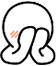P1046 陶陶摘苹果

### 输入输出样例

#### 输入

100 200 150 140 129 134 167 198 200 111
110

5

### 说明/提示

NOIP2005 普及组第一题

#include "stdio.h"
int main()
{
int a = {0}, x, y = 0;
for (int i = 0; i < 10; i++)
scanf("%d", &a[i]);
scanf("%d", &x);
for (int i = 0; i < 10; i++)
{
if (a[i] <= x + 30)
y++;
}
printf("%d", y);
return 0;
}

P1047 校门外的树

### 输出格式

1个整数，表示马路上剩余的树的数目。

### 输入输出样例

500 3
150 300
100 200
470 471

#### 输出

298

NOIP2005普及组第二题一开始没想明白。。。借鉴的代码

#include "stdio.h"
int main()
{
int a, b, c, d, e, f=0;
scanf("%d%d", &b, &c);
for (int i = 0; i <= b; i++)
{
a[i] = 1;//把有树的标记上
}
for (int j = 0; j <= c - 1; j++)
{
scanf("%d%d", &d, &e);
for (int k = d; k <= e; k++)
{
a[k] = 0;//被砍的记得改为0
}
}
for (int l = 0; l <= b; l++)
{
if (a[l] == 1)
f++;//统计剩余的树
}
printf("%d", f);
return 0;
}

P1427 小鱼的数字游戏

### 输入输出样例

#### 输入

3 65 23 5 34 1 30 0

#### 输出

30 1 34 5 23 65 3

#include "stdio.h"
int main()
{
int a,i;
for (i = 0;; i++)
{
scanf("%d", &a[i]);
if (a[i] == 0)
break;
}
for (i=i-1; i >= 0; i--)
{
printf("%d ", a[i]);
}
return 0;
}

P1428 小鱼比可爱

6
4 3 0 5 1 2

0 0 0 3 1 2

### 说明/提示

#include "stdio.h"
int main()
{
int n, a, b = {0}, i, j, out = 0;
scanf("%d", &n);//输入鱼的数目
for (i=0; i < n; i++)
scanf("%d", &a[i]);
for (i = 0; i < n; i++)
{
for (j = 0; j < i; j++)
{
if (a[i] > a[j])
b[i]++;
}
}
for(i=0;i<n;i++)
printf("%d ", b[i]);
return 0;
}

P2141 珠心算测验

### 题目描述

(本题目为2014NOIP普及T1)

### 输入输出样例

4
1 2 3 4

2

#### 说明/提示

【样例说明】

【数据说明】

#include "stdio.h"
int main()
{
int n, a, flag = {0}, i, j, out = 0;
scanf("%d", &n);
for (i=0; i < n; i++)
scanf("%d", &a[i]);
for (i = 0; i < n; i++)
{
for (j = i+1; j < n; j++)
{
for (int k = 0; k < n; k++)
{
if (a[i] + a[j] == a[k] && k != i && k != j&&flag[k]==0)
{
out++;
flag[k] = 1;
}

}
}
}
printf("%d",out);
return 0;
}

P1567 统计天数

### 输出格式

1 行：一个整数，表示最高气温一直上升的最长连续天数。

### 输入输出样例

#### 输入

10
1 2 3 2 4 5 6 8 5 9

#### 输出

5

#include "stdio.h"
int main()
{
long int N, a = {0}, z = 1, out = 1;
scanf("%ld", &N);
for (int i = 0; i < N; i++)
scanf("%ld", &a[i]);
for (int i = 0; i < N; i++)
{
if (z > out)
out = z;
if (a[i] > a[i - 1])
z++;
else
z = 1;
}
printf("%ld", out);
return 0;
}